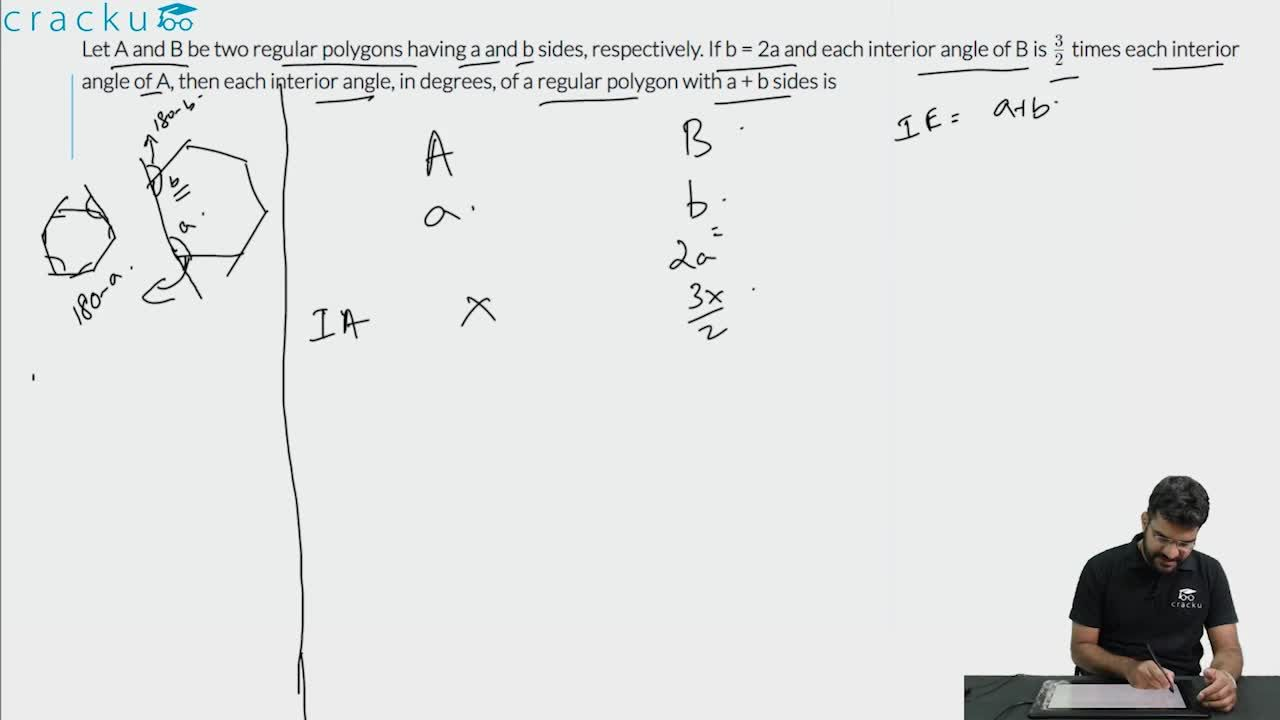Question 97

# Let A and B be two regular polygons having a and b sides, respectively. If b = 2a and each interior angle of B is $$\frac{3}{2}$$ times each interior angle of A, then each interior angle, in degrees, of a regular polygon with a + b sides is

Solution

Each interior angle in an n-sided polygon = $$\frac{\left(n-2\right)180\ }{n}$$

It is given that each interior angle of B is $$\frac{3}{2}$$ times each interior angle of A and b = 2a

$$\frac{\left(b-2\right)180\ }{b}$$ = $$\ \frac{\ 3}{2}\times\$$ $$\frac{\left(a-2\right)180\ }{a}$$

$$2\times\ \left(b-2\right)\times\ a\ =\ 3\times\ \left(a-2\right)\times\ b$$

2(ab-2a) = 3(ab-2b)

ab-6b+4a=0

a*2a-12a+4a=0

$$2a^2-8a=0$$

a(2a-8) = 0

a cannot be zero so 2a=8

a=4, b = 4*2=8

a+b = 12

Each interior angle of a regular polygon with 12 sides = $$\ \frac{\ \left(12-2\right)\times\ 180}{12}$$

=150

### View Video Solution